Sunday 25th July 2021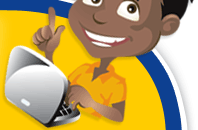# Classroom Key Stage 2 / KS2 - Maths Activities & Resources

## Welcome to the Maths section of the Key Stage 2 classroom. Within KS2 Maths you will find online @school assessment tests that will help children to enhance their learning and revise the subject of Maths at Key Stage 2 level.

•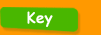•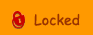••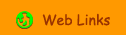•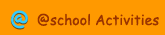•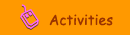•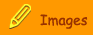###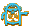Shape & Space

Angles - Level 6 - Test about calculating angles for level 6 children.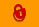Angles - Level 6 - 2 - Test about calculating angles for level 6 children.Angles - Level 6 - 3 - Test about calculating angles for level 6 children.Area - Interactive test about matching up different areas.Area - Level 6 - 2 - Interactive test to calculate the area of two squares.Area - 2 - Interactive test to match up different areas.Area - 3 - Interactive test to match up different areas.Area - Level 6 - Test about the area of a shape for level 6 children.Centimetre Cubes - Interactive test to calculate the volume of a cuboid.Circle and Triangle - Level 6 - Interactive test to work out the area of a triangle and the remaining circle.Clockwise Dial - Interactive activity to find various angles on a dial.Co-ordinates - Interactive test to find various co-ordinates on a graph.Cubes - Level 6 - Interactive test to solve the problem of the volume and ratio of cubes.Equilateral Triangles - Interactive test about equilateral triangles.Isosceles Triangle - Interactive test to work out the co-ordinate of an isosceles triangle.Mirror Line - Interactive test to find the correct reflection for a given shape.Pentagon - Interactive test about right angles and pentagons.Shaded Shape - Interactive test to find the symmetrical line of a shape and an angle.Shape Grid - Online test about shapes in a grid.Square Grid - 1 - Interactive test to find the area of a shape by finding its percentage.Square Grid - 2 - Interactive test about 2D shapes on a grid.